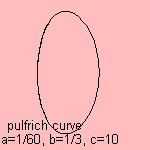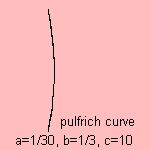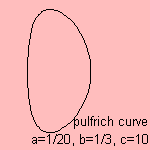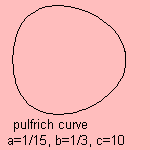# Nickalls-Pulfrich curve

## higher

last updated: 2005-06-19The Pulfrich effect is an optical illusion in which an object which is moving in a plane parallel to the viewer's forehead seems to move out of that plane and to approach (or recede from) the viewer. To achieve this illusion it is necessary only to dim the light reaching one eye, by a filter. The illusion is stereoscopic: it only occurs when using both eyes.
The classical way to demonstrate the effect is using a swinging pendulum.
The phenomenon is due to a unilateral increase in visual latency, resulting from the decrease in retinal image intensity due to the filter.

Now we try to catch the phenomenon in a mathematical equation:
Now imagine that a vertical rod, moving in a horizontal circle on a turntable, is viewed horizontally from the side with a filter in front of one eye. It was Pulfrich (1922) who described some of the illusions that are to be seen while the turntable is slowing down. This slowing down makes studying of the phenomenon very difficult, that's why Dick Nickalls chose to observe a situation with constant angular velocity and a view from various distances.
He found the theoretical apparent curves of the rod, and verified them experimentally 1), as function of:

• the latency as result of the filter
• the distance from the rod to the center of the turntable
• the distance between the two eyes
• the distance form the observer to the center of the turntable

These parameters are related as follows to the constants in the given formula:

• 2a is the latency angle (latency difference in seconds * angular velocity)
• 2b is the distance between the eyes / distance from the rod to the center of the turntable
• c is the distance between the observer and the center of the turntable / distance from the rod to the center of the turntable

When varying the latency, and keeping the distances the same, the rod appears to have the same (nearby) or the opposite (far away) rotation direction of the turntable. In the transition situation the curve is an arc of a circle. Beneath five situations are given with latency angle growing from right to left.It has been found in astronomy that observers differ consistently in observational measurements. Each observer has a so-called personal equation factor, explained as differences in reaction time and visual illusion as the Pulfrich effect.

This should mean that the magnitude of the Pulfrich effect is personal (should this not be the case for all optical illusions?), and e.g. a function of age.

notes

1) RWD Nickalls: the rotating Pulfrich effect, and a new method of determining visual latency differences, Vision Research, Vol 26, pp 367-372, 1986.
While working on the Pulfrich experiments a generalized conic theorem was found, to be read in the Mathematical Gazette (2000), vol. 84 (July), pp 232-241.